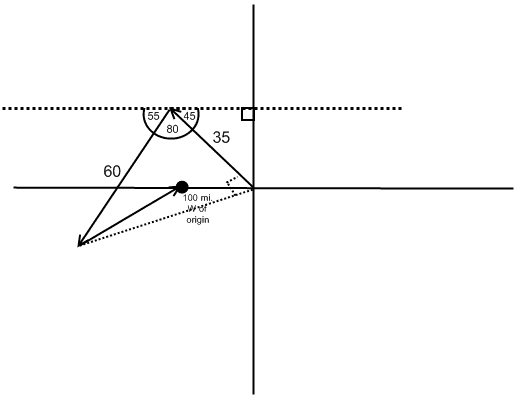# What must the third displacement be?

• Rker

## Homework Statement

A delivery man travels 35 mi northwest to his first stop, 60 mi in a direction 35° west of south to his second stop. His third stop is supposed to be 100 mi west of his original position. What must his third displacement be (magnitude and direction) for him to reach his destination?

?

## The Attempt at a Solution

I drew this inaccurate graph.I'd rather not make an accurate graph for these kind of problems since I don't always have the resources to do so. I'm familiar with SOHCAHTOA, but I'm not sure how to use it to find the solution to this problem.

This is what someone suggested for a similar problem, but I'm having trouble understanding it.

- Displacement: 3.5km north, 5km west and 1.7km south gives a net distance of
√[(3.5 - 1.7)² + 5²] (Pythagorean theorem) = √28.24
to the direction of north-west (not exactly northwest)
- Direction: This is more trigonometric than geometric but whatever.
Let α be the angle between "East" axis and the direction of movement, we have:
tanα = y/x = (3.5 - 1.7)/5 = 0.36 => α = arctan(0.36)

Let the starting point be O. the firsat stop be A, second stop be B and third stop be C.
In triangle OAC, OA, OC and angle AOC is given.Using cosine rule you can find AC, and usind sine rule you can find angle OAC. Repeat the same thing for the triangle CAB.

That does make things easier to understand, but I'm not even certain that I calculated the angles correctly since my graph is inaccurate.

Once I do find the angles for triangle OAC, how will that help me find the angles for CAB?

Angle OAB is known.
CAB = OAB - OAC.

Is angle OAB 80 degrees? I tried redrawing the graph on paper, and now I don't think it is.

35 degrees west of south means 55 degrees south of west.
So 55 + 45 + θ = 180 degrees.

Oh, okay.

Also, when I redrew the graph more accurately, point C actually turned up being positioned more west than point B.